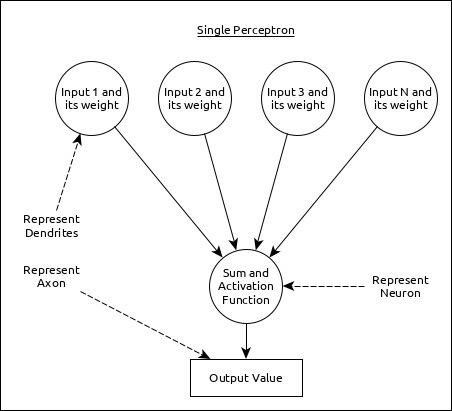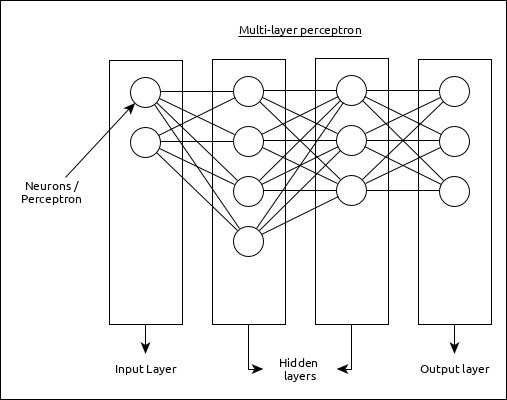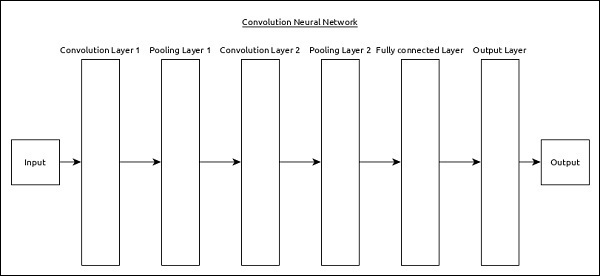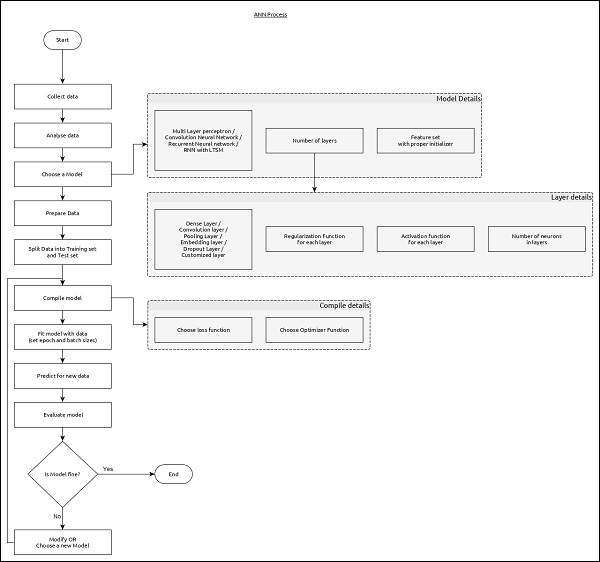# Keras - Overview of Deep learning

Deep learning is an evolving subfield of machine learning. Deep learning involves analyzing the input in layer by layer manner, where each layer progressively extracts higher level information about the input.

Let us take a simple scenario of analyzing an image. Let us assume that your input image is divided up into a rectangular grid of pixels. Now, the first layer abstracts the pixels. The second layer understands the edges in the image. The Next layer constructs nodes from the edges. Then, the next would find branches from the nodes. Finally, the output layer will detect the full object. Here, the feature extraction process goes from the output of one layer into the input of the next subsequent layer.

By using this approach, we can process huge amount of features, which makes deep learning a very powerful tool. Deep learning algorithms are also useful for the analysis of unstructured data. Let us go through the basics of deep learning in this chapter.

## Artificial Neural Networks

The most popular and primary approach of deep learning is using “Artificial neural network” (ANN). They are inspired from the model of human brain, which is the most complex organ of our body. The human brain is made up of more than 90 billion tiny cells called “Neurons”. Neurons are inter-connected through nerve fiber called “axons” and “Dendrites”. The main role of axon is to transmit information from one neuron to another to which it is connected.

Similarly, the main role of dendrites is to receive the information being transmitted by the axons of another neuron to which it is connected. Each neuron processes a small information and then passes the result to another neuron and this process continues. This is the basic method used by our human brain to process huge about of information like speech, visual, etc., and extract useful information from it.

Based on this model, the first Artificial Neural Network (ANN) was invented by psychologist Frank Rosenblatt, in the year of 1958. ANNs are made up of multiple nodes which is similar to neurons. Nodes are tightly interconnected and organized into different hidden layers. The input layer receives the input data and the data goes through one or more hidden layers sequentially and finally the output layer predict something useful about the input data. For example, the input may be an image and the output may be the thing identified in the image, say a “Cat”.

A single neuron (called as perceptron in ANN) can be represented as below −Here,

• Multiple input along with weight represents dendrites.

• Sum of input along with activation function represents neurons. Sum actually means computed value of all inputs and activation function represent a function, which modify the Sum value into 0, 1 or 0 to 1.

• Actual output represent axon and the output will be received by neuron in next layer.

Let us understand different types of artificial neural networks in this section.

## Multi-Layer Perceptron

Multi-Layer perceptron is the simplest form of ANN. It consists of a single input layer, one or more hidden layer and finally an output layer. A layer consists of a collection of perceptron. Input layer is basically one or more features of the input data. Every hidden layer consists of one or more neurons and process certain aspect of the feature and send the processed information into the next hidden layer. The output layer process receives the data from last hidden layer and finally output the result.## Convolutional Neural Network (CNN)

Convolutional neural network is one of the most popular ANN. It is widely used in the fields of image and video recognition. It is based on the concept of convolution, a mathematical concept. It is almost similar to multi-layer perceptron except it contains series of convolution layer and pooling layer before the fully connected hidden neuron layer. It has three important layers −

• Convolution layer − It is the primary building block and perform computational tasks based on convolution function.

• Pooling layer − It is arranged next to convolution layer and is used to reduce the size of inputs by removing unnecessary information so computation can be performed faster.

• Fully connected layer − It is arranged to next to series of convolution and pooling layer and classify input into various categories.

A simple CNN can be represented as below −Here,

• 2 series of Convolution and pooling layer is used and it receives and process the input (e.g. image).

• A single fully connected layer is used and it is used to output the data (e.g. classification of image)

## Recurrent Neural Network (RNN)

Recurrent Neural Networks (RNN) are useful to address the flaw in other ANN models. Well, Most of the ANN doesn’t remember the steps from previous situations and learned to make decisions based on context in training. Meanwhile, RNN stores the past information and all its decisions are taken from what it has learnt from the past.

This approach is mainly useful in image classification. Sometimes, we may need to look into the future to fix the past. In this case bidirectional RNN is helpful to learn from the past and predict the future. For example, we have handwritten samples in multiple inputs. Suppose, we have confusion in one input then we need to check again other inputs to recognize the correct context which takes the decision from the past.

## Workflow of ANN

Let us first understand the different phases of deep learning and then, learn how Keras helps in the process of deep learning.

### Collect required data

Deep learning requires lot of input data to successfully learn and predict the result. So, first collect as much data as possible.

### Analyze data

Analyze the data and acquire a good understanding of the data. The better understanding of the data is required to select the correct ANN algorithm.

### Choose an algorithm (model)

Choose an algorithm, which will best fit for the type of learning process (e.g image classification, text processing, etc.,) and the available input data. Algorithm is represented by Model in Keras. Algorithm includes one or more layers. Each layers in ANN can be represented by Keras Layer in Keras.

• Prepare data − Process, filter and select only the required information from the data.

• Split data − Split the data into training and test data set. Test data will be used to evaluate the prediction of the algorithm / Model (once the machine learn) and to cross check the efficiency of the learning process.

• Compile the model − Compile the algorithm / model, so that, it can be used further to learn by training and finally do to prediction. This step requires us to choose loss function and Optimizer. loss function and Optimizer are used in learning phase to find the error (deviation from actual output) and do optimization so that the error will be minimized.

• Fit the model − The actual learning process will be done in this phase using the training data set.

• Predict result for unknown value − Predict the output for the unknown input data (other than existing training and test data)

• Evaluate model − Evaluate the model by predicting the output for test data and cross-comparing the prediction with actual result of the test data.

• Freeze, Modify or choose new algorithm − Check whether the evaluation of the model is successful. If yes, save the algorithm for future prediction purpose. If not, then modify or choose new algorithm / model and finally, again train, predict and evaluate the model. Repeat the process until the best algorithm (model) is found.

The above steps can be represented using below flow chart −